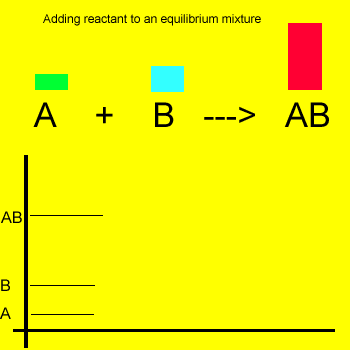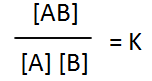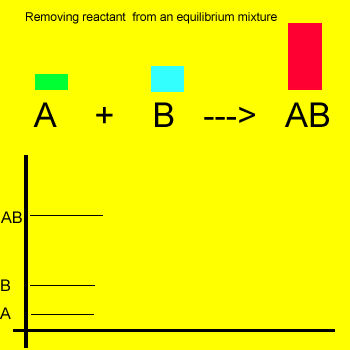Equilibrium Le Chatelier's Principle If the temperature of the system remains constant then the equilibrium position of the reaction will shift to counter any changes made. Le Chatelier's Principle is a useful generalisation that states " If an equilibrium system is subjected to a change the system will adjust itself to partially oppose the effect of the change". Lets see some examples. The mixture of reactant and product molecules are at equilibrium. The straight line on the graph indicates the mixture is at equilibrium. Adding extra amount of reactant "A" forces the reaction forward in attempt to remove the extra amount of reactant. As a consequence the amount of reactant "B" reduces while the amount of product increases. As the reaction shifts to the right the equilibrium expression below remains constant.Removal of a substance from the equilibrium mixture forces the reaction to shift so as to try and form the substance that was removed or decreased in amount. In the equilibrium mixture on the left reactant "B" is reduced. The reaction therefore moves to the left to compensate for the reduction and maintain a constant value for the equilibrium mixture. As a result the amount of reactant "A" increases while the amount of product "AB" decreases. The new equilibrium mixture will have the same equilibrium constant as previously. ONLY temperature can change the equilibrium constant. At the same temperature therefore, the equilibrium constant will stay the same no matter what changes are made to the equilibrium mixture. Exercise Continue